Modelling and Simulation of the SVC for Power System Flow Studies: Electrical Network in voltage drop

Narimen Aouzellag Lahaçani, Boubekeur Mendil

Department of Electrical Engineering, Department of Electronic

Faculty of Science and Engineering, A. Mira University, 06000 Bejaia, Algeria

Email(s): nlahacani@yahoo.fr

Abstract

The goal of any Flexible AC Transmission Systems (FACTS) devices study is to measure their impact on the state of the electrical networks into which they are introduced. Their principal function is to improve the static and dynamic properties of the electrical networks and that by increasing the margins of static and dynamic stability and to allow the power transit to the thermal limits of the lines.

To study this impact, it is necessary to establish the state of the network (bus voltages and angles, powers injected and forwarded in the lines) before and after the introduction of FACTS devices. This brings to calculate the powers transit by using an iterative method such as Newton-Raphson. Undertaking a calculation without the introduction of FACTS devices followed by a calculation with the modifications induced by the integration of FACTS devices into the network, makes it possible to compare the results obtained in both cases and thus assess the interest of the use of devices FACTS.

Keywords

SVC device, Newton-Raphson method, power flow, voltage regulation, Matlab.

Introduction

The wide-area networks, with increased transfers of power, became very complex to manage and because of its limitations, the coordination of the control and adjustment systems can pose problems. To solve the problems of powers flow, the Flexible Alternating Current Systems Transmission (FACTS) devices  offer an alternative to the traditional means of regulation. These devices based on the power electronics, make it possible to control the voltage level and/or the power transit in the electrical network lines. The FACTS devices can be used for the control of the active power as well as for that of the reactive power or the voltage. The study of the FACTS led to providing models containing nodal admittance matrix which allow representing, study and simulating FACTS devices and their behaviours in an electrical network. These models were applied to elaborate a program in MATLAB language.

Presence of the FACTS in the network

To study the impact of the introduction of Static Var Compensator (SVC) device into a network, changes in the nodal admittances matrix and in the Jacobian matrix  will be made. Initially, the introduction of the FACTS is carried out by modification of the admittances matrix, and this by, varying the control parameter of the FACTS and by observing the changes on the bus voltages and the transit powers in the lines. This allows deducing the best value which allows an ideal compensation of the network. The modification of the placement  of the FACTS in the network makes it possible to choose the best place in which to introduce the device in the studied network. The second stage consists in fixing a consign value (of voltage or of power) for which a program in MATLAB language will find the value of the control size. This requires modifications of Jacobian matrix to introduce the parameter control as a variable. This way, it will check, if it is possible to regulate a size (voltage or power) to a value consign without deteriorating the static performances of the network.

Construction of the nodal admittance matrix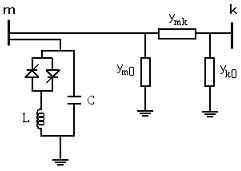Figure 1: SVC inserting in a line

SVC placed at the beginning of line

For an SVC connected at a bus bar m of a line section represented by the quadruple (ym0, ymk, yk0) as shown in figure 1, the contribution of the SVC   to the new admittance matrix relates to the element shunt. It results in the admittance matrix of the line.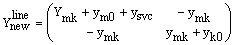(1)

Such as: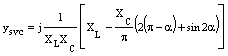(2)

The SVC reactance is given as the following expression:(3)

SVC placed at the middle of a line

When the static compensator is inserted into the middle of a line, the latter is divided into two identical sections. The SVC is connected to the additional bus “t” as illustrated by figure 2.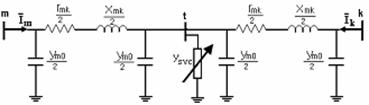Figure 2: SVC placed at the middle of a line

In order to take into account this new bus, an additional line and a column should be added to the nodal admittance matrix. To avoid having to change the number of buses of the network and thus the admittance matrix size, a transformation star-delta makes it possible to reduce the system by removing the bus “t” and by calculating the parameters of an equivalent line. The figure 3 illustrates the steps to obtain this equivalent line.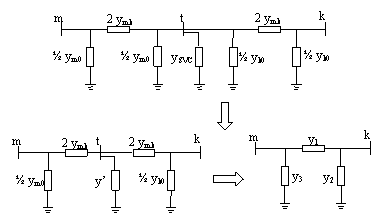Figure3: Y-∆ transformation

Such as:(4)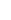All the admittance matrix elements of a line with an SVC in its middle are modified, such as: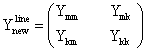(5)(6)

Power flow calculation

Insertion of an SVC at the beginning of a line

It results from these modifications on the nodal admittances matrix and from the Jacobian matrix . These modifications are detailed in what follows.

We modelled the SVC as being a variable transverse admittance which is connected to a bus m of the network. Thus, the effect of this, is based only on the modification of the element y in the admittances matrix. The new modified matrix is written as follows:(7)

This new matrix is used to calculate the new power transit. By varying the firing angle of the SVC “α”, it is possible to plot the curves of voltages variation at the buses. They allow to locate the best point of compensation of the network (all voltage drops within the limits =+0.05). The variation curves of the powers losses in the lines can be obtained according to “α” which makes it possible to measure the impact of the SVC device on these lines. These curves will be studied for the compensation of a network in the voltage drop case.

Modification of the Jacobian matrix

To take into account the introduction of the SVC as a voltage regulator and thus to allow the simulation program to calculate the ideal firing angle to maintain the bus voltage where it is inserted to a consign value, it is necessary to introduce modifications into the Jacobian matrix.

When the SVC is inserted at a bus, this is controlled, and thus its voltage is maintained in magnitude with a fixed value; this makes it possible to eliminate the term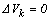(such as k is the controlled bus index). This term is substituted the difference “α” which will allows to have, after convergence, the firing angle of the thrusters which allows the maintenance of the consign voltage. The matrix system becomes then as follows:(8)

But knowing that: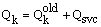(9)

Then: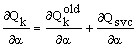(10)

and as Qk depends only on the firing angle “α”, it results :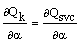(11)

With,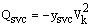, then: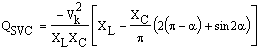(12)

Finally, the expression (10 ) becomes: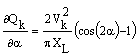(13)

Insertion of an SVC in the middle of a line

An SVC inserted in the middle of a line of the network, conducts to modifications of the admittances nodal matrix and Jacobian matrix which are detailed as follows.

According to the equation (6), the elements Ymm, Ykk, Ymk, Ykm will be modified in such a way as to produce the new following matrix of admittances:(14)

With,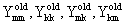being the elements of the matrix before the introduction of the SVC(15)

The same case studies will be carried out as for an SVC at the beginning of line. The results obtained will be compared for the two cases of placements; and conclude if an SVC in middle of a line can ensure the compensation with the same effectiveness as two SVC placed in ends of line.

The studied network structure

The simulation results are obtained for a network test shown in figure 4, whose buses are numbered from 1 to 5, and the lines are numbered from 1 to 7.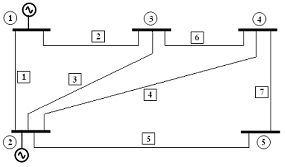Figure 4: The studied network structure

The network data are grouped in tables 1 and 2.

Table 1: Buses data

 Bus V (pu) δ (°) Generation Load P (pu) Q(pu) P(pu) Q(pu) 1 1.000 0.000 0.000 0.000 0.000 0.000 2 1.020 0.000 0.400 0.000 -0.200 -0.100 3 1.000 0.000 0.000 0.000 -0.450 -0.150 4 1.000 0.000 0.000 0.000 -0.400 -0.050 5 1.000 0.000 0.000 0.000 -0.600 -0.100

Table 2: Lines data

 Line R (pu) X (pu) B (pu) 1-2 0.0200 0.0600 0.0600 1-3 0.0800 0.2400 0.0600 2-3 0.0600 0.1800 0.0400 2-4 0.0600 0.1800 0.0400 2-5 0.0400 0.1200 0.0300 3-4 0.0100 0.0300 0.0200 4-5 0.0800 0.2400 0.0600

The network state (voltages and powers) after convergence is given in tables 3, 4 and 5.

Table 3: Voltage magnitudes at the buses (pu)

 1 2 3 4 5 1 1.02 0.9711 0.9712 0.9673

Table 4: Voltage angles at the buses (deg)

 1 2 3 4 5 0 -3.3941 -5.7398 -6.1192 -7.0576

Table 5: transit powers in the lines

 N° P (pu) Q (pu) 1 0.8968 -0.2997 2 0.4064 -0.0199 3 0.2482 0.0622 4 0.2808 0.0527 5 0.5503 0.0908 6 0.1873 -0.0722 7 0.0626 -0.0281

Insertion of the SVC in the network

To observe the impact of the presence of a SVC in the network, a state study of the network was made, in the event of voltage drop in a node. The localisation of the SVC in the network was changed to deduce the one which offers the best compensation, and to observe the voltages variation of at the buses according to the reactive power injected by the SVC, this will give the ideal firing angle for the network compensation.

The SVC parameters used in calculations are given in table 6.

Table 6: SVC Parameters

 Xc  (pu) XL (pu) αmin (°) αmax (°) 1.0700 0.2880 90 180

The figure 5 shows the SVC admittance variation according to firing angle α.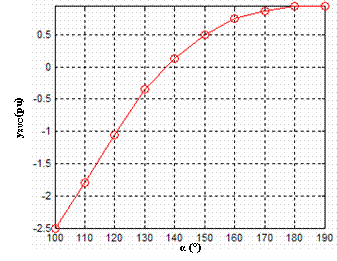Figure 5: SVC admittance according to firing angle α.

Network in voltage drop

In this case, node 4 is overloaded in such a way as to cause the voltage drop in this bus and those adjacent. The modifications go on the active P and reactive Q powers, which take the values 1.40 pu and 0.40 pu respectively. It results a change in the network state. The results obtained are detailed in tables 7, 8 and 9.

Table 7: Voltage magnitudes at the buses (pu)

 1 2 3 4 5 1 1.02 0.9434 0.9344 0.9676

Table 8: Voltage angles at the buses (deg)

 1 2 3 4 5 0 -6.5862 -10.747 12.1209 -11.0652

Table 9:power losses in the lines (pu)

 N° ∆P (pu) ∆Q (pu) 1 0.0693 0.1467 2 0.0454 0.0889 3 0.0182 0.0161 4 0.0270 0.0428 5 0.0221 0.0368 6 0.0059 0.0000 7 0.0017 -0.0400

To solve the problem of this voltage) drop, an SVC is introduced successively at buses 3 and 4, and then in the middle of the line 6 which connects them.

Insertion of an SVC at a bus 4

The principle is to vary “α” from 100° until 180° to observe the effect of the SVC on the bus voltages and the power losses in the lines. Figures 6 and 7 show the influence of the firing angle on the voltage magnitudes like on the voltage angles.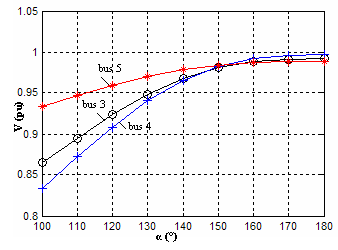Figure 6: Voltage variation at the buses according to α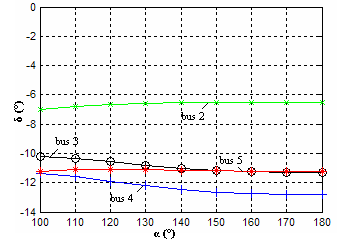Figure 7: Angle variation at the buses according to α

Interpretation

The figure 6 shows that the voltages are compensated starting from a value “α” of 140° (equivalent to QSVC of 0,7084). The voltages starting from this value are within the tolerated limits (±5%). Therefore, the SVC fulfils its role of voltage regulator as well as it makes it possible to reduce the losses in the lines as shown in figures 8 and 9.  Indeed, the SVC makes it possible to fill by a contribution of local reactive energy the consumption at the level of the overloaded bus, and thus, to reduce the request for reactive energy from the generator and the assessment bus. This naturally makes it possible to reduce the power forwarded through the line (especially that connected to bus 4) and thus by rebound the losses.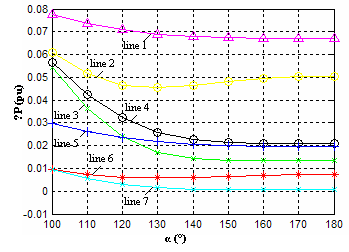Figure 8: Active losses in lines according to α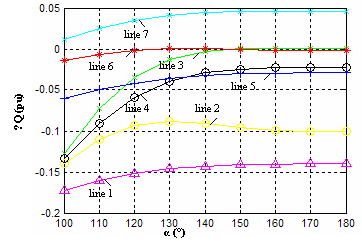Figure 9: Reactive losses in lines according to α

Insertion of an SVC at a bus 3

The principle is to vary α from 100° until 180° in order to observe the effect of the SVC on the bus voltage and the power losses in the lines. Figures 10 and 11 show the influence of the firing angle of the SVC on the voltage magnitudes as well as on the voltage angles.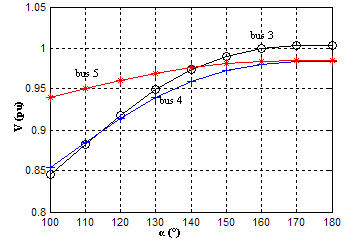Figure 10: Voltage variation at the buses according to α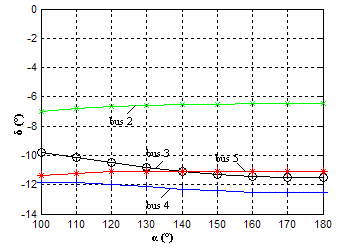Figure 11: Angle variation at the buses according to α

Interpretation

The figure 10 shows the voltages variation at the buses according to the firing angle of the SVC. It shows well that starting from 140°, all the bus voltages are within the limits ±5%. The SVC manages to compensate for the voltage drop introduced by the overload of bus 4, and this for the reactive power value equal to 0,4486 pu. This proves that even if introduced at a different bus than the overloaded bus, the SVC manages to compensate for the voltage drop just by inserting it in a bus close to the overloaded bus.

Figures 12 and 13 show the influence of the insertion of the SVC on the active and reactive losses in the lines and that according to the angle of firing. The losses decrease with the increase in the level of compensation, this is explained by the fact that the reactive energy injected by the SVC makes it possible to fill the need for consumption at the level of buses 3 and 4, and thus, it decreases the powers forwarded towards these buses starting from the assessment bus (1) and the generator (2). With the reduction in the reactive powers forwarded in the lines, it results in a decrease of the losses (in lines 3 and 6).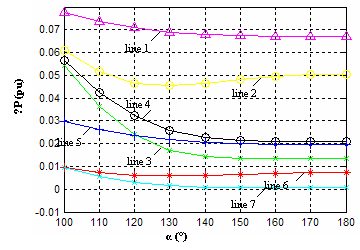Figure 12: Active losses in lines according to α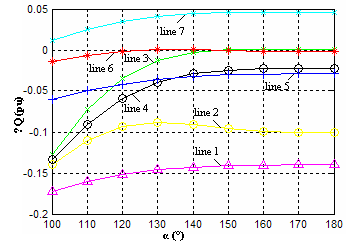Figure 13: Reactive losses in lines according to α

Comparison between an SVC placed at the middle of a line and an SVC placed at the buses located at the ends of this line

In order to study the influence of the placement in the line of the SVC on the voltage regulation, two SVC are placed respectively at buses 3 and 4, and then in the middle of line 6 which connects these two buses, and thus, compare their performances for the voltages compensation at buses 3,4 and 5. Figures 14 and 15 show the voltage magnitudes variation and voltage angles at the buses according to the firing angle “α”, and thus, assess the reactive power injected by the SVC/SVCs for the different placements. The uninterrupted curves correspond to two SVC placed at buses 3 and 4, while the curves in dotted line show the effect of an SVC inserted in the middle of line 6.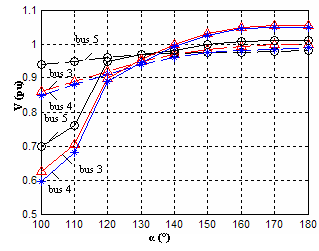Figure 14: Voltage magnitudes according to α for various placements (SVC at the buses, SVC in the middle of the line 6)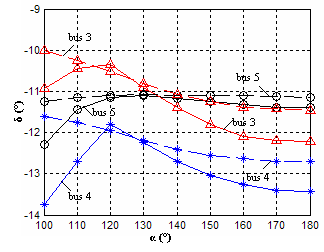Figure 15: Voltage angles according to α for various placements (SVC at the buses, SVC in the middle of the line 6)

Interpretation

The figure 14 shows that the SVC in the middle of the line offers almost the same performances as the two SVC connected at the ends. In fact, the voltages at buses 3, 4 and 5 reach the threshold of 0,95pu which with a firing angle of 120° (correspondent to a QSVC value of approximately 0,7084pu) for the two cases. Concerning the two SVC connected to the buses, the program does not converge for values lower than a firing angle of 110° (which corresponds to a QSVC value of 0,8572pu). Figures 16 and 17 show the importance of the SVC devices insertion to improving the powers forwarded in the line 6.

Powers transmitted in line 6

To assess the choice effect of the SVC, figures 16 and 17 represent the evolution of active and reactive powers forwarded in the line 6. Three comparative curves are given representing a network without FACTS, with an SVC placed at the middle of the line and with two SVC at the ends of this line.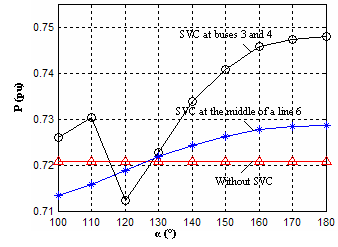Figure 16: Transmitted reactive powers in line 6 according to α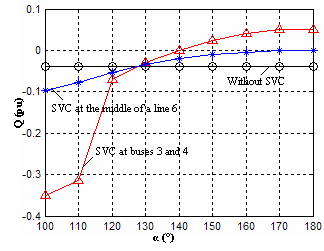Figure 17: Transmitted reactive powers in line 6 according to α

A comparison of the three curves on each figure shows that the presence of the SVC devices allows the increase in the power forwarded in the line.  Two SVC inserted at the ends of the line provide better results compared to a single SVC connected to the middle of the line, but it should be noted that for values lower than QSVC equal to 0,8572pu,  the method of calculation does not converge.

Conclusion

The study undertaken on case regarding the state of the tested network (voltage drop), showed the influence of the SVC device insertion in the electrical network. The effectiveness of the SVC to compensate the voltage drop which is due to an inductive overload at one of the network buses were checked.

An SVC device allows indeed restoring a good level of bus voltages after an imbalance even if it is not inserted into the overloaded bus. Indeed, we showed that if the SVC is connected to an adjacent bus to the overload, it has also good performances. The SVC allows also, even though it is not its first vocation, to reduce the powers losses in the lines of the compensated network, and thus, increase the power transfers.

Then, in order to study the influence of the placement on the SVC performances, a comparison was made on the placement of two SVC connected to two buses, with only one SVC inserted in the middle of the line which connects these two buses. The results obtained by the simulation program show that one SVC in the middle of the line offers almost the same performances for the maintenance of the voltage level as two SVC devices assembled at the buses.

As a conclusion, an SVC installed in the middle of the line is more profitable (cost effectiveness) as a solution for the maintenance of a good voltage profile, but due to the installation cost of SVC devices as they require the installations construction of the switchyard type, the owners/users of the networks prefer to install SVC devices in already existing stations.

References

1.      M. Crappe and S. Dupuis: “ Les systèmes flexibles de transport de l’énergie électriques”, Stabilité et sauvegarde des réseaux électriques , Chapitre 4, pp 149-204, Paris, Hermes, 2003

2.      N.G. Hingoran, “High power electronics and flexible AC transmission system”, IEEE power engineering review, pp 3-4, July 1988

3.      E. Acha, C.R. Fuerte-Esquivel, H. Ambriz-Perez and C. Angeles-Camacho: “FACTS, modelling and simulation in power networks”,Chichester, Angland: John Wiley and Sons, 2004

4.      G. T. Heydt , Douglas J. Gotham: “Power flow control and power flow studies for systems FACTS devices”,  IEEE Transactions on Power Systems, Vol. 13, No. 1, February 1998

5.      B. Sookananta, S. Galloway, G. M. Burt and J. R. McDonald, “The placement of FACTS devices in modern electrical network”, Proceedings of the 41st International, UPEC '06, Vol.2,  pp.780-784, 2006

6.      G. Radman, R.S. RAJE “Power flow model/calculation for power systems with multiple FACTS controllers”, Elsevier, Electrical power systems research,2006

7.      J.A. Domnguez-Navarro, J.L . Bernal-Agustin, A. Diaz, D. Requena, E.P. Vargaz Emilio “Optimal parameters of FACTS devices in electric power systems applying evolutionary strategies”, Electrical Power and Energy Systems vol. 29, no1, pp. 83-90, 2007